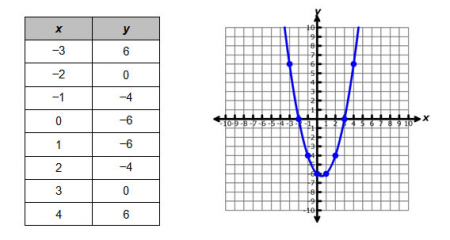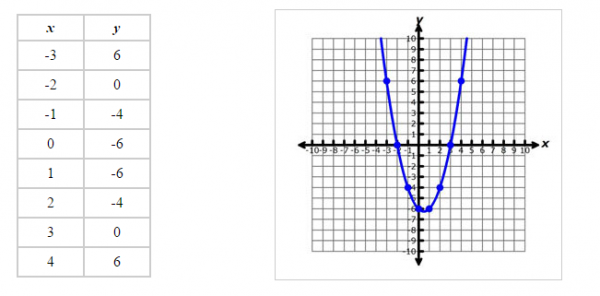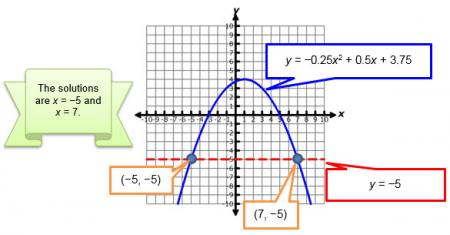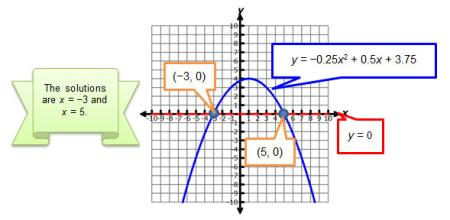Let's Get Started

Let's explore how to solve quadratic equations by looking at their graphs.

TEKS Standards and Student Expectations

A(1) Mathematical process standards. The student uses mathematical processes to acquire and demonstrate mathematical understanding. The student is expected to:

A(1)(C) select tools, including real objects, manipulatives, paper and pencil, and technology as appropriate, and techniques, including mental math, estimation, and number sense as appropriate, to solve problems

A(1)(D) communicate mathematical ideas, reasoning, and their implications using multiple representations, including symbols, diagrams, graphs, and language as appropriate

A(8) Quadratic functions and equations. The student applies the mathematical process standards to solve, with and without technology, quadratic equations and evaluate the reasonableness of their solutions. The student formulates statistical relationships and evaluates their reasonableness based on real-world data. The student is expected to:

A(8)(A) solve quadratic equations having real solutions by factoring, taking square roots, completing the square, and applying the quadratic formula

Resource Objective(s)

Given a quadratic equation, the student will use graphical methods to solve the equation.

Essential Questions

How can you solve a quadratic equation by looking at the graph?

How do know how many solutions a quadratic equation has?

Vocabulary

Introduction

Let's investigate ways to use a graph to represent and determine the solution to a quadratic equation.

The graph and table below show points for the quadratic function y = x² − x − 6.Both the graph and the table of values can be used to solve the equation x² − x – 6 = 0 which is related to the function y = x² − x − 6. You can find when y = 0 on the table, and the x-value at that point will solve the function. The x-value where the graph crosses the x-axis can also be used to solve a quadratic equation. You will use similar thinking to locate points on graphs that satisfy particular conditions.

Using Graphs to Describe Solutions

Although all quadratic functions have a solution, the solution may not always be a real number. Therefore, a quadratic function may have two real roots, one real root, or zero real roots.

Examine the three graphs below. Click the plus sign by the Start button to learn more about their solutions.

Using Graphs to Determine Solutions

A graph is the picture of the points that make a function true. For example, the graph and table below show points for the quadratic function y = x² – x – 6.Both the graph and the table of values can be used to solve the equation x² – x – 6 = 0, which is related to the function y = x² – x – 6.  Click on each question to check your answer.

Interactive:  In this interactive, the graphs represent equations related to the function
y = x² – x – 6. The red line represents the graph y = k. Match each graph with the equation by dragging the graph to the appropriate slot. Then indicate the correct solutions represented in the graph by dragging the correct ordered pair(s) to the solution box.

Video

Source
Evaluating Functions Using a Graphing Calculator, Gdawy Enterprises, You Tube

Practice

Determine the solution to the each quadratic equation by graphing. Click on the problem to see the answer.

Summary

A graph is a useful representation for determining the solution of a quadratic equation. To best use a graph, think about a quadratic equation as written in two parts: ax² + bx + c = k

Graph each part of the quadratic equation: ax² + bx + c = k and y = k

Look for the intersection of the two graphs. The x-coordinates of the intersection points will tell you the values of x that are solutions to the original equation.

For example, the graph of f(x) = −0.25x² + 0.5x + 3.75 is shown below. You can use the graph to determine the solutions to the related equation −0.25x² + 0.5x + 3.75 = -5.You can also use the graph to solve the equation -0.25x² + 0.5x + 3.75 = 0 by identifying the x-intercept since the x-axis is the same as the line y = 0.